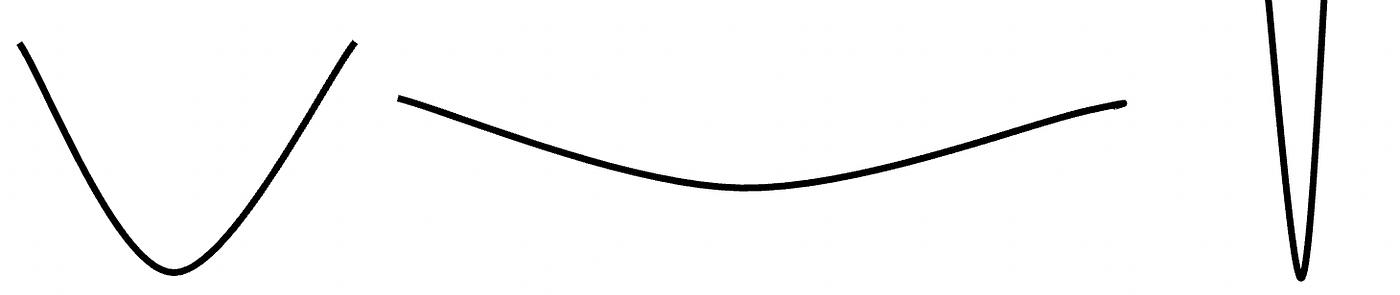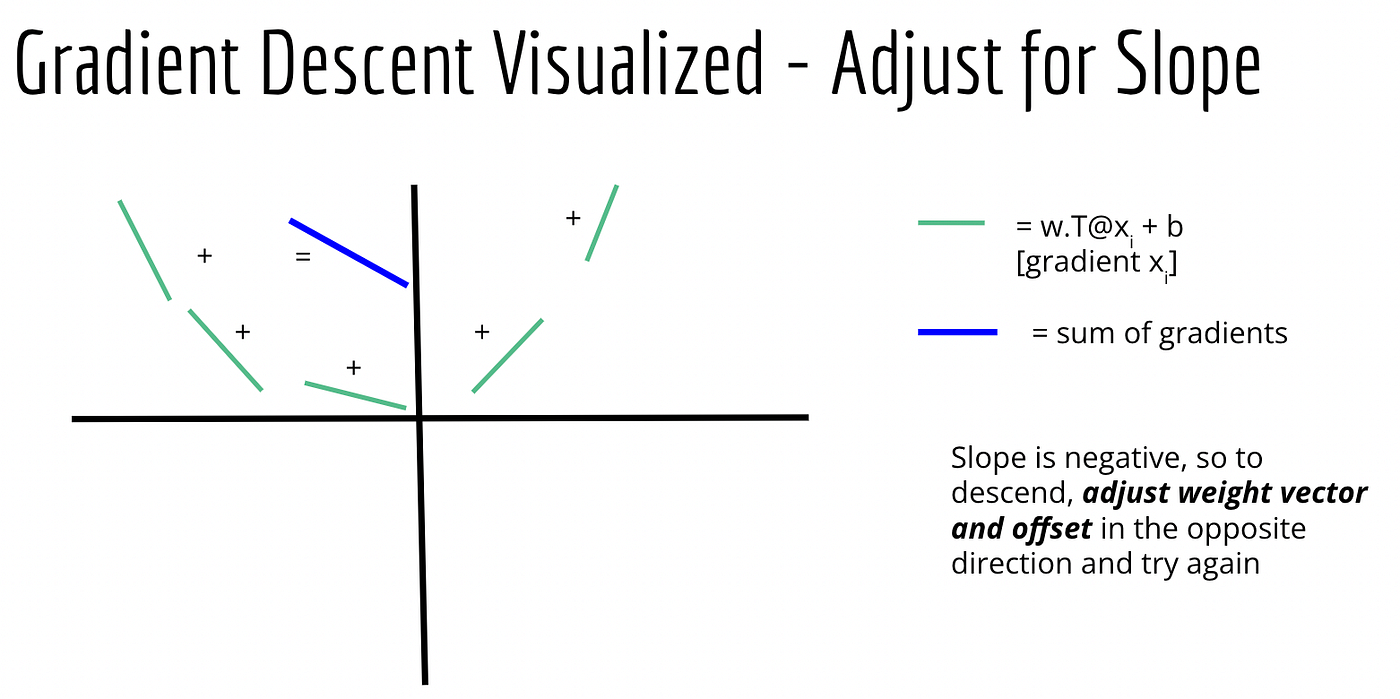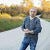# Marble in a Steel Bowl Part 2

Gradient Descent Concepts Further Defined

So you wanted a data science post? Well, here’s your data science post. :)

When I last posted about gradient descent, I compared it conceptually to dropping a marble in a steel bowl. The bowl itself is invisible, so you don’t know where the bottom is. However, you do know that if the marble hits the bottom or close to it, it’s going to bounce straight back up. If not, the marble will hit the side and bounce in the direction of the center. Once you observe the direction of the bounce, you adjust your position in that direction and drop the marble again, and keep doing this until the marble bounces close enough to straight back up for you to call the center as being reached.

All in all, a pretty decent analogy.

What I want to do in this post is to formalize some of the math concepts that go into gradient descent and make it work, and then explain how — in a pure numbers world — you know which way the marble bounces (and thus, the direction you need to move to get closer to the bottom of the bowl), how you figure out how far to move, and so forth.

Let’s start with a basic question: how do we know we’re dealing with a bowl in the first place? If the shape is invisible, couldn’t it really be anything, including a shape with multiple local minimums — i.e. various false bottoms? Well, it turns out with logistic regression and gradient descent, we actually start by making an assumption about our data labels, that they are sigmoidal in nature, which is a fancy mathematical way of saying they’re pretty clearly one label or another, such that few if any of the labels are going to fall along a decision boundary where a given label is equally probable to be one thing or the other. If we accept this assumption, we can furthermore assume our function has a convex shape with a single minimum error point. The problem then is that we have no idea what type of convex shape it is or where it exists in coordinate space.Sure, these are all convex shapes, but the math is going to be very, very different between the three.

If you’ll hearken back to the discussion on Naive Bayes as a linear classifier, we actually did the exact opposite of this. We looked at the distribution of our labels and then based the probability / likelihood of a data point falling on one side or the other of a classification line based on an assumption about the distribution of the data. We assumed our data were normally distributed (although other assumptions can be made and tested to see if they predict better) and so given a known set of labels, you could predict what a label of an unknown point would be based on that assumption.

With logistic regression, we don’t make assumptions about our data — we use every data point, but assume a distribution of our labels (sigmoid) such that the probability of an unknown label y is determined based on the totality of all of the data with an assumed convex shape of the label distribution. Again, it’s taking what we did with Naive Bayes and flipping it exactly on its head — making assumptions about the label distribution rather than the data distribution. If you don’t have a lot of data, not a great approach, so Naive Bayes would be preferable. However, given enough data, this approach can produce superior outcomes compared to making guesses about how data is distributed. (Normal distributions just aren’t, well, all that normal…)

So back to gradient descent then — first off, gradient is another way of saying “slope,” so if you remember basic geometry and that slope equals rise over run (or change in y over change in x, in a two-dimensional space), you’ve got that basic idea of what a gradient is. In two dimensional space, it’s just a line defined by a formula wx + b (again, basic geometry here — although you probably learned it as mx+b instead of wx+b.) In matrix math / numpy notation that turns into w.T@x + b. If you are defining gradients of points that make up a convex shape, you start by making a guess about what w and b values should be (usually you give them all 0 values before the first update — almost certainly going to be wrong…), and then you find the slope/gradient of the tangent of every single data point you have using those values. This slope can be calculated by finding the first derivative of the sigmoid function given each data point (which goes beyond what I’ll discuss in this post) but the bottom line is this is a knowable formula that can be coded decently easily.

Then comes the fun part — if you have 100 data points, you have calculated 100 gradients/slopes. You simply sum up all of the gradients, and check out the slope of the line / hyperplane that results. Based on this aggregate slope, you adjust your w and b values in the opposite direction, and then recalculate all of the gradients again with those new values.The slope is how you determine which way the marble bounced when you dropped it.

You repeat this process until one of two things happens — either you come up with an aggregate gradient/slope that’s close enough to zero to call the bottom reached, or if you have limited resources or time, you set a certain number of iterations and just call it done after those number of iterations have been run.

One big thing I’ve left out up to this point — how do you know how much to adjust your w and b values at each iteration? The unfortunate answer is “you don’t.” You know the direction, but the bowl is still invisible so you don’t know the magnitude. You just have to guess at how much adjustment to make, plain and simple. One way to do this is by using a method known as Taylor’s expansion, in which you take your w and adjust it by adding the gradient of w (or more precisely, the negative gradient, since gradients by definition ascend, so for “descent” you do the opposite) times some alpha value.

This alpha value is kind of arbitrary, but the wrong one can have a big impact. Set it too small, and your w adjustments are very small — you are only making tiny movements towards the center of the bowl every time. Sure, you’re getting closer and closer to the bottom, but you’re moving so slowly that it might take forever for you to get there. On the other hand, set it too large, and you are making big moves in the direction of the bounce — possibly past the bottom of the bowl and over to the other side. Your adjustment risks bouncing first off one side, then the other, then back on the other side, and on and on without ever having a chance of actually hitting the bottom, just changing the direction of your bounce at each iteration. That’s also not good.

One way to approach this is to start with a relatively large alpha value — say, 1, for example, and then at each iteration make it smaller and smaller. That way, in the beginning you are taking pretty large steps, but as you get closer to the center, you are taking smaller and smaller steps so as not to overshoot too far. The adjustment for offset b at each iteration follows a similar pattern. Again, once you have reached a w and b value such that the sum of the gradients (negative gradients) of all of your data points approaches zero, you have found the right w and b values to apply to your validation and test data and make predictions.

And that, folks, comprises the theory behind gradient descent. Make an assumption about the distribution of your data labels (rather than the data), then use that assumption and find the slope of the resulting label distribution such that you can approximate the bottom of the convex shape it makes.

--

--## Jason Eden

Data Science & Cloud nerd with a passion for making complex topics easier to understand. All writings and associated errors are my own doing, not work-related.